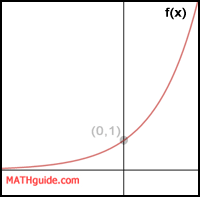Exponential Functions: Translations
View the Lesson | MATHguide homepage Updated July 17th, 2022Directions:
Use the graph of f(x) to the right to determine the translation:

g(x) = 3(2)x - 5 - 2.

Translation Statement for g(x):

 “The exponential function f(x) = 2x must be shifted Select 1 2 3 4 5 6 7 8 units to the Select right left and Select 1 2 3 4 5 6 7 8 units Select up down andvertically stretched by a factor of Select 1 2 3 4 5 6 .”

Characteristics of g(x), to the nearest hundreth:

The domain is (-∞, +∞).
The range is (, +∞).
The asymptote is = .
The y-intercept is (, ).
The point (0,1) on f(x) shifts to (, ) on g(x).